The Theory of patches-vector
10th November 2015
liamoc.net

Recently I released the Haskell library patches-vector, a library for simple, but theoretically-sound manipulation of patches, or diffs, on a "document", which in this case just consists of a Vector of arbitrary elements.

I approached the development of this library from a formal perspective, devising laws for all the operations and rigorously checking them with QuickCheck. I defined a patch as a series of edits to a document, where an edit is simply an insertion, deletion, or replacement of a particular vector element.

newtype Patch a = Patch [Edit a] deriving (Eq)

data Edit a = Insert  Int a
| Delete  Int a
| Replace Int a a
deriving (Show, Read, Eq)

We have a function, apply, that takes a patch and applies it to a document:

apply :: Patch a -> Vector a -> Vector a

Patches may be structurally different, but accomplish the same thing. For example, a patch that consists of Delete and an Insert may extensionally be equivalent to a patch that does a single Replace, but they are structurally different. To simplify the mathematical presentation here, we define an equivalence relation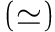that captures this extensional patch-equivalence: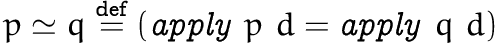## Documents and patches form a category

To define further operations, we must first note that patches and documents form a category. A category is made up of a class of objects (in this case documents), and a class of arrows or morphisms (in this case patches). For each object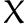, there must be an identity morphism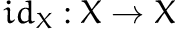, and for each pair of morphisms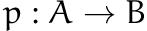and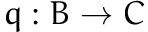there must be a composed morphism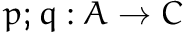. They must satisfy the following laws:

• Left-identity: for any morphism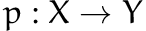,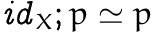• Right-identity: for any morphism,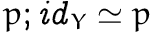• Associativity: for any three morphisms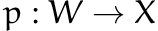,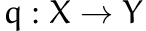,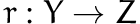,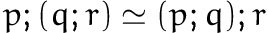The category laws laws comprise the first part of our specification. Translating it into Haskell, I made Patch a an instance of Monoid, just for convenience, even though the composition operator is not defined for any arbitrary patches, and therefore patches technically are not a monoid in the algebraic sense.

Then, the above laws become the following QuickCheck properties:

forAll (patchesFrom d) $\a -> a <> mempty == a forAll (patchesFrom d)$ \a -> mempty <> a == a

forAll (historyFrom d 3) $\[a, b, c] -> apply (a <> (b <> c)) d == apply ((a <> b) <> c) d Here, patchesFrom d is a generator of patches with domain document d, and historyFrom d 3 produces a sequence of patches, one after the other, starting at d. ## Our category is indiscrete In the case of patches and documents, they form what's called indiscrete category or a chaotic category, as there exists a single, unique1 patch between any two documents. A function to find that patch is simply diff, which takes two documents and computes the patch between them, using the Wagner-Fischer algorithm (Wagner & Fischer 1974). diff :: Eq a => Vector a -> Vector a -> Patch a It's easy to come up with correctness properties for such a function, just by examining its interaction with the identity patch, the composition operator, and apply: apply (diff d e) d == e diff d d == mempty apply (diff a b <> diff b c) a == apply (diff a c) a ## Our category is a groupoid As there exists a patch between any two documents, it follows that for every patchthere exists an inverse patch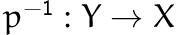such that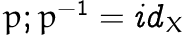and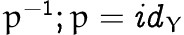. We define a function, inverse, in Haskell: inverse :: Patch a -> Patch a And we can check all the usual properties of inverses: forAll (patchesFrom d)$ \p -> p <> inverse p == mempty

forAll (patchesFrom d) $\p -> inverse p <> p == mempty forAll (patchesFrom d)$ \p -> inverse (inverse p) == p

forAll (patchesFrom d) $\p -> inverse mempty == mempty forAll (historyFrom d 2)$ \[p, q] ->
inverse (p <> q) == inverse q <> inverse p

We can also verify that the inverse patch is the same that we could have found by diff:

apply (diff d e) d == apply (inverse (diff e d)) d

A category that contains inverses for all morphisms is called a groupoid. All indiscrete categories (such as patches) are groupoids, as the inverse morphism is guaranteed to exist. Groupoids are very common, and can also be thought of as a group2 with a partial composition relation, but I find the categorical presentation cleaner.

## Merges are pushouts

So, we have now specified how to compute the unique patch between any two documents (diff), how to squash patches together into a single patch (composition), how to apply patches to a document (apply), and how to compute the inverse of a given patch (inverse). The only thing we're missing is the crown jewel of patch theory, how to merge patches when they diverge.

I came to patch theory from concurrency control research, and not via the patch theory of Darcs (Jacobson 2009), so there are some differences in how I approached this problem compared to how Darcs does.

In their seminal paper (Ellis & Gibbs 1989) on the topic, Ellis and Gibbs define a function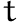that, given a diverging pair of patches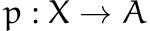and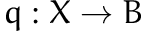, will produce new patches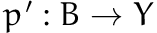and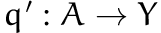, such that the result of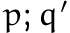and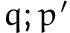is the same:They called this approach operational transformation, but category theory has a shorter name for it: a pushout. A pushout of two morphismsandconsists of an object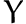and two morphismsandsuch that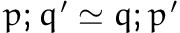. The pushout must also be universal, but as our category is indiscrete we know that this is the case without having to do anything.

We can use this pushout, which we call transform, as a way to implement merges. Assuming a document history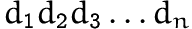and an incoming patch from version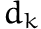, where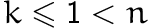, we can simply transform the input patch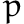against the composition of all the patches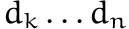, resulting in a new patch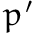that can be applied to the latest document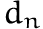.

Note that just specifying the transform function to be a pushout isn't quite sufficient: It would be perfectly possible to resolve two diverging patchesand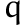by using patches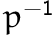for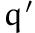and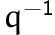for, and they would resolve to the same document, but probably wouldn't be what the user intended.

Instead, our transform function will attempt to incorporate the changes ofintoand the changes ofinto, up to merge conflicts, which can be handled by a function passed in as a parameter to transform:

transformWith :: (a -> a -> a) -> (Patch a, Patch a) -> (Patch a, Patch a)

Then we can add the pushout property as part of our QuickCheck specification:

forAll (divergingPatchesFrom d) $\(p,q) -> let (p', q') = transformWith const p q in apply (p <> q') d == apply (q <> p') d If the merge handler is commutative, then so is transformWith: forAll (divergingPatchesFrom d)$ \(p,q) ->
let (p' , q' ) = transformWith (*) p q
(q'', p'') = transformWith (*) q p
in p' == p''
&& q' == q''

We can also ensure that transformWith keeps the intention of the input patches by using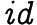as one of the diverging patches:

forAll (patchesFrom d) $\ p -> transformWith (*) mempty p == (mempty, p) forAll (patchesFrom d)$ \ p ->
transformWith (*) p mempty == (p, mempty)

## Coda

And with that, we've specified patches-vector. A patch theory is "just" a small, indiscrete groupoid with pushouts3. We can theoretically account for all the usual patch operations: inversion, composition, merging, diff, and apply, and this gives rise to a spec that is rock solid and machine-checked by QuickCheck.

The full code is available on GitHub and Hackage. Please do try it out!

I also wrote a library, composition-tree (also on Hackage and GitHub), which is similarly thoroughly specified, and is a convenient way to store a series of patches in a sequence, with good asymptotics for things like taking the mconcat of a sublist. I use these two libraries together with pandoc, acid-state and servant to make a basic wiki system with excellent support for concurrent edits, and edits to arbitrary versions. The wiki system is called dixi (also on GitHub and Hackage).

I independently invented this particular flavour of patch theory, but it's extremely similar to, for example, the patch theory underlying the pijul version control system (see Mimram & Di Giusto 2013), which also uses pushouts to model merges.

Another paper that is of interest is the recent work encoding patch theory inside Homotopy Type Theory using Higher Inductive Types (Angiuli et al. 2014). HoTT is typically given semantics by ∞-groupoids, so it makes sense that patches would have a natural encoding, but I haven't read that paper yet.

Also, another paper (Swierstra & Löh 2014) uses separation logic to describe the semantics of version control, which is another interesting take on patch theoretic concepts.

Angiuli, C., Morehouse, E., Licata, D. R., & Harper, R. (2014). Homotopical patch theory. In Proceedings of the 19th ACM SIGPLAN international conference on functional programming, New York, NY, USA: ACM, pp. 243–256.
Ellis, C. A., & Gibbs, S. J. (1989). Concurrency control in groupware systems. In Proceedings of the 1989 ACM SIGMOD international conference on management of data, New York, NY, USA: ACM, pp. 399–407.
Jacobson, J. (2009). A formalization of darcs patch theory using inverse semigroups, UCLA Computational and Applied Mathematics.
Mimram, S., & Di Giusto, C. (2013). A categorical theory of patches. Electronic Notes in Theoretical Computer Science, 298, 283–307.
Swierstra, W., & Löh, A. (2014). The semantics of version control. In Proceedings of the 2014 ACM international symposium on new ideas, new paradigms, and reflections on programming & software, New York, NY, USA: ACM, pp. 43–54.
Wagner, R. A., & Fischer, M. J. (1974). The string-to-string correction problem. J. ACM, 21(1), 168–173.

1. Up to, of course.↩︎

2. A group is a monoid with inverses.↩︎

3. What's the problem?↩︎